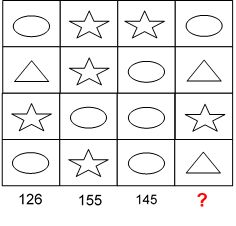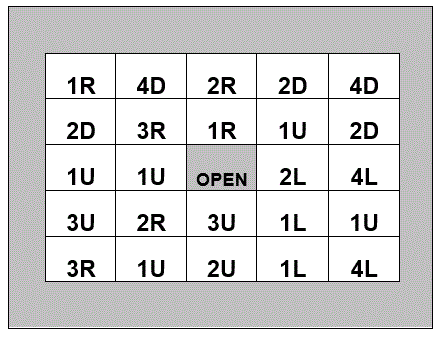Select Page

Each month, a new set of puzzles will be posted.  Come back next month for the solutions and a new set of puzzles, or subscribe to have them sent directly to you.

## MIND-Xpander numeric puzzles

1. The dimensions of one box are all two-thirds of the dimensions of another. If the volume of the smaller is 64 cubic centimetres, what is the volume of the larger box?
2. Arrange the digits 1, 2, 3, 4 and 5, using only plus & minus signs (addition & subtraction), so that they will total 111. Using the same instructions, arrange the digits for totals of 222, and 333. (Note: There may be more than one solution)

## EQUATE-Sums puzzle

Can you work out what each of the four symbols are worth? The totals of the three columns are given. Can you also work out the missing total?## EQUATE- TakeAway puzzle

The missing numbers are between 1 & 9. The three numbers in top columns, when subtracted by the lower two numbers, equals the three totals along the bottom, and the three numbers in the left rows, when subtracted by the right two numbers, equals the three totals at the far right. The top left number, when subtracted by the lower two numbers on its diagonal, equals the total in the bottom, far right box. With one number provided, solve the puzzle by finding all missing numbers that satisfy all the results as shown.## Feedback

There are more than one way of doing these puzzles and may well be more than one answer.  Please let me and others know what alternatives you find by commenting below.  We also welcome general comments on the subject and any feedback you'd like to give.

If you have a question that needs a response from me or you would like to contact me privately, please use the contact form.

## Get more puzzles!

If you've enjoyed doing the puzzles, consider ordering the book; 150+ of the best puzzles in a handy pocket sized format. Click here for full details.

## MIND-Xpander numeric puzzles

1. If QC = 14, TJ=10 & OK = 4, what would HA = ? Solution: The numeric order of the 1st letter in the alphabet (where H=8) minus that of the 2nd letter (where A=1) gives value of 7.
2. What do you get if you divide 40 by a half and add 20 to the result? Solution: (40 ÷ 1/2) + 20 = (40 x 2/1) + 20 = 100
3. How can you mathematically combine six 8’s to make 1000? Solution: (888 – 88) ÷ .8 = 1000

## Safety-code logic puzzle

To open this safe you must press all the buttons in the correct order. The number on each button tells you how many squares to move, and the letters tells the direction (U = up, D = down, L = left and R = right). Which is the first button you must press to open this safe?

Solution: Start at ‘1R’ button in 3rd column, 2nd row## DARTS numeric puzzle

Using 4 darts and the following square dart board, find all combinations to score exactly 150. Numbers/boxes can be used more than once. All darts must be used, and each dart must be within one of the numbered boxes. (Note: there are several correct solutions.)Solutions

113 + 13 + 12 + 12 = 150

111 + 16 + 13 + 10 = 150

108 + 16 + 16 + 10 = 150

93 + 33 + 12 + 12 = 150

89 + 33 + 16 + 12 = 150

87 + 43 + 10 + 10 = 150

79 + 49 + 12 + 10 = 150

54 + 43 + 43 + 10 = 150